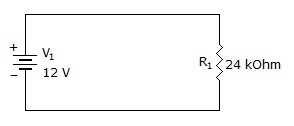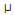# Electronics - Resistance and Power

### Exercise :: Resistance and Power - General Questions

11.

What current is flowing in the circuit?A. 288 kA B. 2 kA C. 50 mA D. 500A

Explanation:

No answer description available for this question. Let us discuss.

12.

The six basic forms of energy are:

 A. light, sun, magnetic, chemical, electrical, and mechanical B. electrical, mechanical, light, heat, magnetic, and chemical C. electrical, mechanical, sun, heat, chemical, and light D. potential, sun, light, chemical, electrical, and mechanical

Explanation:

No answer description available for this question. Let us discuss.

13.

How much energy is stored if 6.24 x 1018 electrons are stored in 4 volts?

 A. 4 joules B. 1.56 x 1018 electrons C. 1.56 coulombs D. 2.496 x 1019 electrons

Explanation:

No answer description available for this question. Let us discuss.

14.

With Ohm's law:

 A. current is inversely proportional to resistance B. resistance is directly proportional to voltage C. voltage is indirectly proportional to power D. current is directly proportional to resistance

Explanation:

No answer description available for this question. Let us discuss.

15.

Power is defined as:

 A. the rate at which energy is used B. watts C. energy D. the rate at which energy is generated